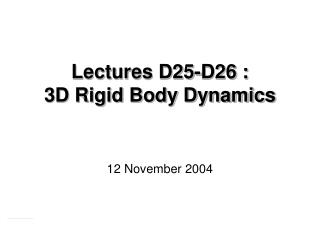DownloadDownload PresentationLectures D25-D26 : 3D Rigid Body Dynamics

# Lectures D25-D26 : 3D Rigid Body Dynamics

Download Presentation## Lectures D25-D26 : 3D Rigid Body Dynamics

- - - - - - - - - - - - - - - - - - - - - - - - - - - E N D - - - - - - - - - - - - - - - - - - - - - - - - - - -
##### Presentation Transcript

1. Lectures D25-D26 :3D Rigid Body Dynamics 12 November 2004

2. Outline • Review of Equations of Motion • Rotational Motion • Equations of Motion in Rotating Coordinates • Euler Equations • Example: Stability of Torque Free Motion • Gyroscopic Motion • Euler Angles • Steady Precession • Steady Precession with M = 0 Dynamics 16.07 1

3. Equations of Motion Conservation of Linear Momentum Conservation of Angular Momentum or Dynamics 16.07 2

4. Equations of Motion in Rotating Coordinates Angular Momentum Time variation • Non-rotating axes XY Z(I changes) big problem! - Rotating axes xyz (I constant) Dynamics 16.07 3

5. Equations of Motion in Rotating Coordinates xyz axis can be any right-handed set of axis, but . . . will choose xyz (Ω) to simplify analysis (e.g. I constant) or, Dynamics 16.07 4

6. Example: Parallel Plane Motion Body fixed axis Solve (3) for ωz, and then, (1) and (2) for Mx and My. Dynamics 16.07 5

7. Euler’s Equations If xyz are principal axes of inertia Dynamics 16.07 6

8. Euler’s Equations • Body fixed principal axes • Right-handed coordinate frame • Origin at: • Center of mass G (possibly accelerated) • Fixed point O • Non-linear equations . . . hard to solve • Solution gives angular velocity components . . . in unknown directions (need to integrate ω to determine orientation). Dynamics 16.07 7

9. Example: Stability of Torque Free Motion Body spinning about principal axis of inertia, Consider small perturbation After initial perturbation M = 0 Small Dynamics 16.07 8

10. Example: Stability of Torque Free Motion From (3) constant Differentiate (1) and substitute value from (2), or, Solutions, Dynamics 16.07 9

11. Example: Stability of Torque Free Motion Growth Unstable Stable Oscillatory Dynamics 16.07 10

12. Gyroscopic Motion • Bodies symmetric w.r.t.(spin) axis • Origin at fixed point O (or at G) Dynamics 16.07 11

13. Gyroscopic Motion • XY Z fixed axes • x’y’z body axes — angular velocity ω • xyz “working” axes — angular velocity Ω Dynamics 16.07 12

14. Gyroscopic Motion Euler Angles Precession – position of xyz requires and – position of x’y’z requires , θand ψ Relation between ( ) and ω,(and Ω ) Nutation Spin Dynamics 16.07 13

15. Gyroscopic Motion Euler Angles Angular Momentum Equation of Motion, Dynamics 16.07 14

16. Gyroscopic Motion Euler Angles become . . . not easy to solve!! Dynamics 16.07 15

17. Gyroscopic Motion Steady Precession Dynamics 16.07 16

18. Gyroscopic Motion Steady Precession Also, note that H does not change in xyz axes External Moment Dynamics 16.07 17

19. Gyroscopic Motion Steady Precession Then, If precession velocity, spin velocity Dynamics 16.07 18

20. Steady Precession with M = 0 constant Dynamics 16.07 19

21. Steady Precession with M = 0 Direct Precession From x-component of angular momentum equation, If then same sign as Dynamics 16.07 20

22. Steady Precession with M = 0Retrograde Precession If and have opposite signs Dynamics 16.07 21